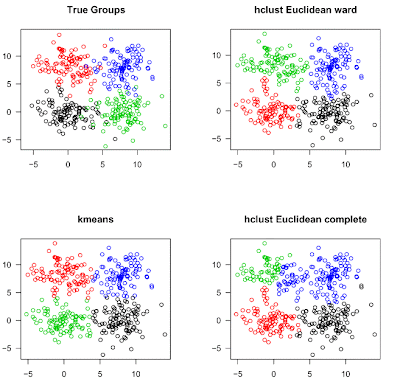## giovedì 10 gennaio 2008

### Hello World for Clustering methods

A hello world program can be a useful sanity test to make sure that the procedure/methods you are analyzing "works" at least for very basic tasks. For this purpose, I create an artificial data set from 4 different 2-dimensional normal distributions to check how well the 4 clusters can be recognized by common clustering methods.

`set1 <- matrix(cbind(rnorm(100,0,2),rnorm(100,0,2)),100,2)set2 <- matrix(cbind(rnorm(100,0,2),rnorm(100,8,2)),100,2)set3 <- matrix(cbind(rnorm(100,8,2),rnorm(100,0,2)),100,2)set4 <- matrix(cbind(rnorm(100,8,2),rnorm(100,8,2)),100,2) dati <- list(values=rbind(set1,set2,set3,set4),classes=c(rep(1,100),rep(2,100),rep(3,100),rep(4,100))) # clustering - common methods op <- par(mfcol = c(2, 2)) par(las =1)plot(dati\$values, col = as.integer(dati\$classes), xlim=c(-6,14), ylim = c(-6,14), xlab="", ylab="", main = "True Groups") party <- kmeans(dati\$values,4)plot(dati\$values, col = party\$cluster, xlab = "", ylab = "", main = "kmeans")hc = hclust(dist(dati\$values), method = "ward")memb <- cutree(hc, k = 4)plot(dati\$values, col = memb, xlab = "", ylab = "", main = "hclust Euclidean ward") hc = hclust(dist(dati\$values), method = "complete") memb <- cutree(hc, k = 4)plot(dati\$values, col = memb, xlab = "", ylab = "", main = "hclust Euclidean complete") par(op)`